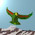## Tuesday, February 22, 2011

### Theory Update 65

We can break up the $21$ outer paths of the tetractys for three qutrits using three trefoil quandles. Recall that the trefoil rules may be expressed in the cyclic form $\{ a \circ b = c, b \circ c = a, c \circ a = b \}$, and this is applied to the multiplication $(123)(456) = ((14)(25)(36))$ on three qudit words. The three quandles are coloured black, red and green.These $21$ paths can be mapped to a $3 \times 3$ planar matrix by interpreting the trefoil cycles as $1$-circulant components.This is just a two qutrit matrix with the first coordinate doubled. There are also three trefoil quandles of the form $\{ XYZ, YYY, ZYX \}$, using the central $6$ path neutrino vertex along with the corner charged lepton vertices. A diagonal element $YYY$ belongs to two trefoil quandles, whereas an off diagonal element $XXY$ belongs to only one.

Note also that the other three trefoil quandles form the $2$-circulant pieces of the above matrix. Each $2$-circulant uses one path of each colour (black, red and green). Thus there are at least $9$ trefoil quandles in the tetractys path set.

1.I hope you escaped effects of the Christchurch earthquake.

2.Physically, yes, but it's too soon to say whether or not all my friends are OK.

3.Glad to see you here too.

With sorrow for Christchurch.

Note: Only a member of this blog may post a comment.